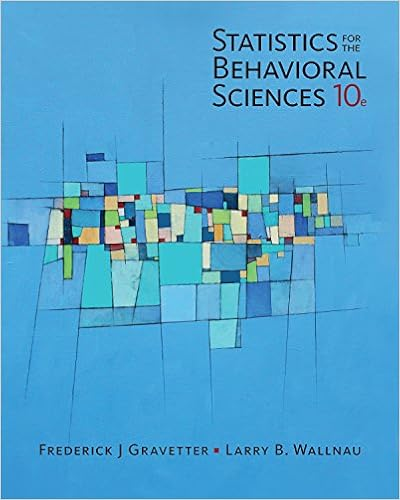# Lecture 10 Chapter 9 - Chapter 9 Introduction to the t...

• Notes
• 20

This preview shows page 1 - 6 out of 20 pages.

##### We have textbook solutions for you!
The document you are viewing contains questions related to this textbook.The document you are viewing contains questions related to this textbook.
Chapter 10 / Exercise 6
Statistics for the Behavioral Sciences
Gravetter/WallnauExpert Verified
Click to edit Master subtitle styleChapter 9 Introduction to thet statisticSpring 2010
##### We have textbook solutions for you!
The document you are viewing contains questions related to this textbook.The document you are viewing contains questions related to this textbook.
Chapter 10 / Exercise 6
Statistics for the Behavioral Sciences
Gravetter/WallnauExpert Verified
Thet StatisticThe t statistic allows researchers to use sample data to test hypotheses about an unknown population mean. Advantage of the t statistic: does not require any knowledge of the population standard deviation. Is used to test hypotheses about a completely unknownpopulation; that is, both μand σare unknown, and the only available information about the population comes from the sample.
The t Statistic (cont.)All that is required for a hypothesis test witht is a sample and a reasonable hypothesis about the population mean. There are two general situations where this type of hypothesis test is used:
The tStatistic (cont.)1.When a researcher wants to determine whether or not a treatment causes a change in a population mean. 2.To compare an actual sample mean with a hypothesized population mean. NOTE: when you know σ, compute a z-score, when you don’t, compute a t-statistic
μ
The Estimated Standard Error and the t Statistic (cont.)The hypothesis test attempts to decide between the following two alternatives:1.Is it reasonable that the discrepancy between M and μis simply due to sampling error and not the result of a treatment effect? 2.Is the discrepancy between M and μmore than would be expected by sampling error alone? That is, is the sample mean significantly different from the population mean?
•••